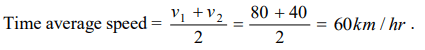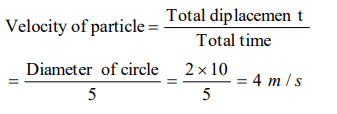## Motion in a Straight Line Questions and Answers Part-2

1. A car travels the first half of a distance between two places at a speed of 30 km/hr and the second half of the distance at 50 km/hr. The average speed of the car for the whole journey is
a) 42.5 km/hr
b) 40.0 km/hr
c) 37.5 km/hr
d) 35.0 km/hr

Explanation:2. One car moving on a straight road covers one third of the distance with 20 km/hr and the rest with 60 km/hr. The average speed is
a) 40 km/hr
b) 80 km/hr
c) $46\frac{2}{3}km/hr$
d) 36 km/hr

Explanation:3. A car moves for half of its time at 80 km/h and for rest half of time at 40 km/h. Total distance covered is 60 km. What is the average speed of the car
a) 60 km / h
b) 80 km / h
c) 120 km / h
d) 180 km / h

Explanation:4. A train has a speed of 60 km/h. for the first one hour and 40 km/h for the next half hour. Its average speed in km/h is
a) 50
b) 53.33
c) 48
d) 70

Explanation:5. Which of the following is a one dimensional motion
a) Landing of an aircraft
b) Earth revolving a round the sun
c) Motion of wheels of a moving trains
d) Train running on a straight track

Explanation: Train running on a straight track

6. A 150 m long train is moving with a uniform velocity of 45 km/h. The time taken by the train to cross a bridge of length 850 meters is
a) 56 sec
b) 68 sec
c) 80 sec
d) 92 sec

Explanation:7. A particle is constrained to move on a straight line path. It returns to the starting point after 10 sec. The total distance covered by the particle during this time is 30 m. Which of the following statements about the motion of the particle is false
a) Displacement of the particle is zero
b) Average speed of the particle is 3 m/s
c) Displacement of the particle is 30 m
d) Both (a) and (b)

Explanation:8. A particle moves along a semicircle of radius 10m in 5 seconds. The average velocity of the particle is
a) $2\pi ms^{-1}$
b) $4\pi ms^{-1}$
c) $2ms^{-1}$
d) $4ms^{-1}$

Explanation:9. A man walks on a straight road from his home to a market 2.5 km away with a speed of 5 km/h. Finding the market closed, he instantly turns and walks back home with a speed of 7.5 km/h. The average speed of the man over the interval of time 0 to 40 min. is equal to
a) 5 km/h
b) $\frac{25}{4} km/h$
c) $\frac{30}{4} km/h$
d) $\frac{45}{8} km/h$